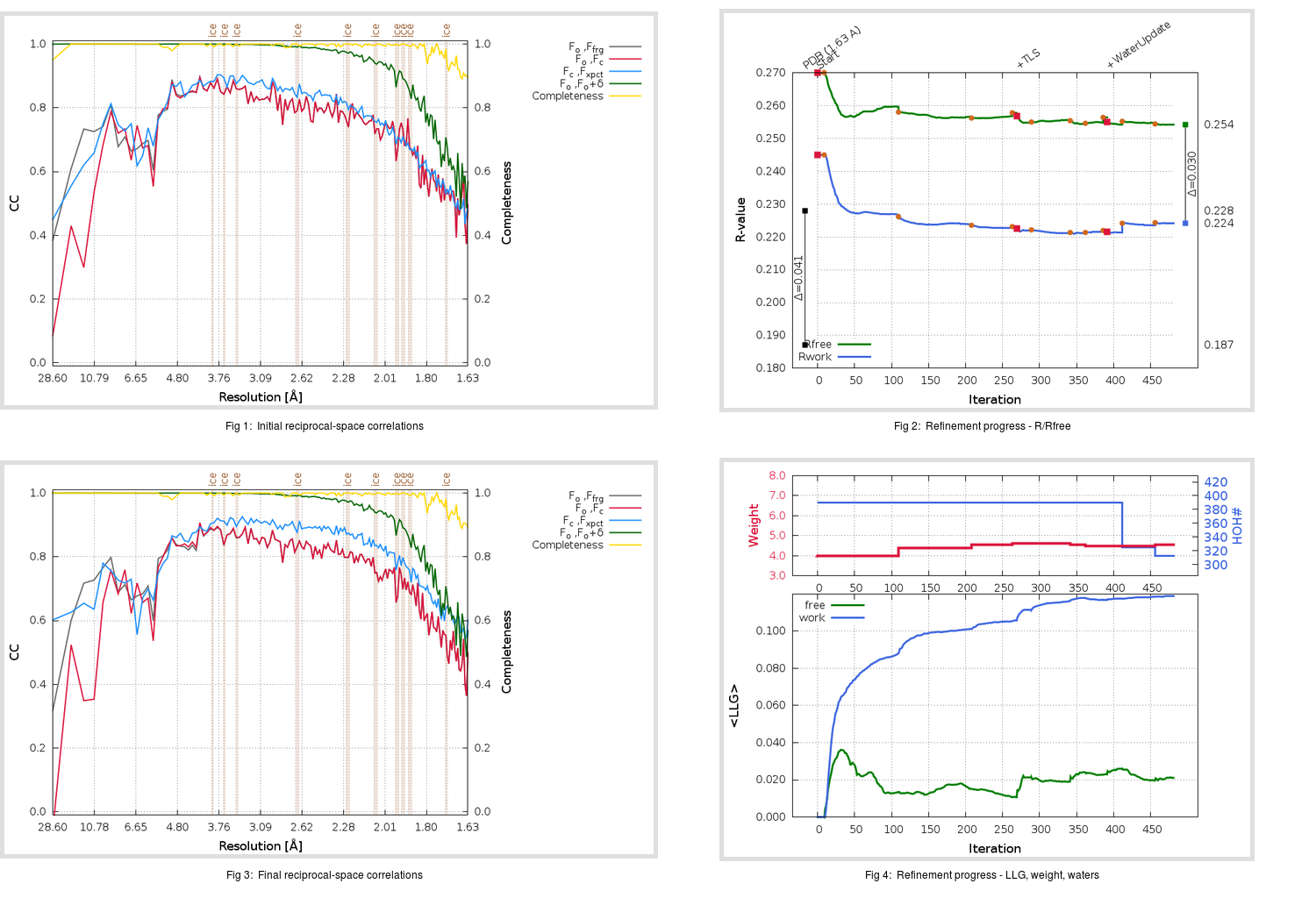Content:

## Deposited

` `
 Date deposited Date data collection Resolution R, Rfree 20200315 20200227 1.63 0.1850 0.2280

Molprobity (CCP4 7.0 version) summary:

```Ramachandran outliers =   0.33 %
favored =  97.68 %
Rotamer outliers      =   1.43 %
C-beta deviations     =     1
Clashscore            =   4.99
RMS(bonds)            =   0.0130
RMS(angles)           =   1.81
MolProbity score      =   1.45
Resolution            =   1.63
R-work                =   0.1850
R-free                =   0.2280
```

```Number of waters      =   390

<B> (all atoms) =   25.35 ( sd =    7.93 ) for       2917 non-hydrogen atoms
<B>   (protein) =   24.26 ( sd =    7.11 ) for       2497 non-hydrogen atoms
<B>     (water) =   34.73 ( sd =   18.03 ) for        390 non-hydrogen atoms
<B>    (others) =   40.63 ( sd =   20.01 ) for         30 non-hydrogen atoms

B min/max       (all non-hydrogen atoms) =   12.48 /  136.15
B min/max   (protein non-hydrogen atoms) =   14.09 /   64.96
B min/max     (water non-hydrogen atoms) =   12.48 /  136.15
B min/max     (other non-hydrogen atoms) =   31.55 /   51.89
```

## BUSTER (re-)refinement

` `

Molprobity (CCP4 7.0 version) summary:

```Ramachandran outliers =   0.33 %
favored =  98.34 %
Rotamer outliers      =   1.43 %
C-beta deviations     =     1
Clashscore            =   3.19
RMS(bonds)            =   0.0111
RMS(angles)           =   1.58
MolProbity score      =   1.23
Resolution            =   1.63
R-work                =   0.2242
R-free                =   0.2541
```

```Number of waters      =   313

<B> (all atoms) =   25.70 ( sd =    8.10 ) for       2840 non-hydrogen atoms
<B>   (protein) =   25.14 ( sd =    7.51 ) for       2497 non-hydrogen atoms
<B>     (water) =   30.04 ( sd =   15.31 ) for        313 non-hydrogen atoms
<B>    (others) =   50.62 ( sd =   27.28 ) for         30 non-hydrogen atoms

B min/max       (all non-hydrogen atoms) =    7.80 /   97.85
B min/max   (protein non-hydrogen atoms) =    7.80 /   48.48
B min/max     (water non-hydrogen atoms) =    8.16 /   79.85
B min/max     (other non-hydrogen atoms) =   24.05 /   97.85
```

Refinement progression:Results:

` `
 File Remark 5REA_aB_refine.01_03_refine.pdb.gz exact refinement commands are in header 5REA_aB_refine.01_03_refine.mtz.gz including original deposited data and several re-refinement map coefficients 5REA_aB_refine.01_03_BUSTER_model.cif.gz including any non-standard compound restraints 5REA_aB_refine.01_03_BUSTER_refln.cif.gz